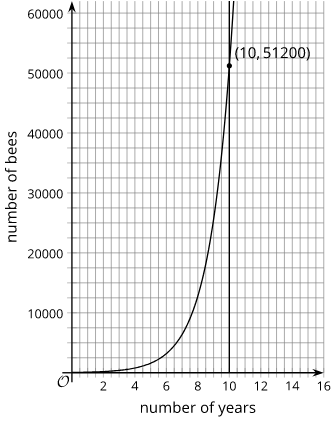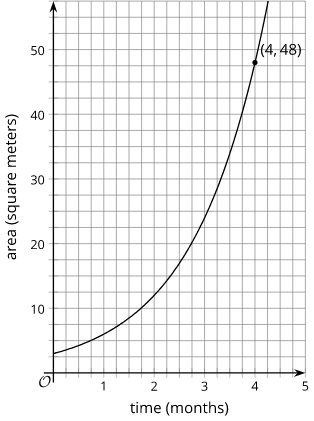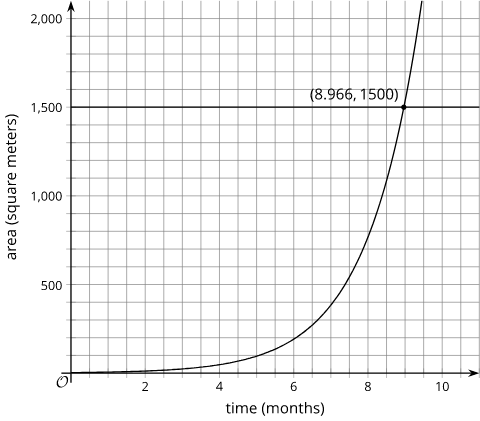# Mathematics Support

Algebra I | Unit 1 2 3 4 5 6 7

## Exponential Functions

### Videos

Main ideas in this unit

In this unit, your student will be introduced to exponential relationships. Earlier, your student studied what mathematicians call linear relationships, where they start with a quantity and add or subtract the same amount repeatedly. In an exponential relationship, you start with a quantity and multiply by the same amount repeatedly.

Exponential relationships are represented by equations in the form $y=a\cdot {b}^{x}$, where $a$ is the quantity you start with, $b$ is the growth factor that you are going to multiply it by, and $x$ is how many times you are going to multiply by $b$. If $b$ is bigger than 1, the amount is growing and if $b$is less than 1, the amount is shrinking. When $b$ is equal to 1, the amount is staying the same.

If you start with 50 bees in your apiary (bee garden), and the number of bees doubles each year, how many bees would you have in 5 years? Let $y$ represent the number of bees and $x$ represent time in years. The starting amount is 50 bees and the multiplier is 2.

While multiplication works well for a situation like this, where we are multiplying by 2 five times, a graph can be a useful tool. If you wanted to know how many bees you would have in 10 years, you could graph $y=50\cdot {2}^{x}$ and see how many bees there are in 10 years.Graphing is especially helpful when looking a long way into the future or when you want to know when something will happen, like when the bee population will reach 1 million.

Florida is having a problem with a toxic green algae that is floating on their waterways, contaminating the water and killing the marine life. Kiran lives on a small lake in south Florida. One day he noticed the algae floating on a 3 square meter area of the lake. A month later, the algae had doubled in size, growing to 6 square meters.

1. If the doubling pattern continues, how many square meters of the lake will be covered in algae in 4 months?
2. If the surface area of the lake is about 1500 square meters, after how many months will the entire lake be covered?

#### Solution

This can be solved using a variety of strategies.

1. You could use a table.

time (months)

area

(square meters)

0 3
1 6
2 12
3 24
4 48

You could write an exponential equation to represent the situation. Let x represent the time in months and y represent the area in square meters.

Substitute 4 for $x$ and solve for $y$.

48 square meters

You could also graph the equation.

$y=3\cdot {2}^{x}$

$y=3\cdot 2\cdot 2\cdot 2\cdot 2=$ 48 square meters

You could also graph the equation.2. Just as with part a, there are several strategies for finding when the algae will cover the whole lake. Extending the graph, adding the graph of $y=1500$, and finding where they intersect is a nice way to find the month.In just under 9 months, the algae will cover 1500 square meters of the lake.